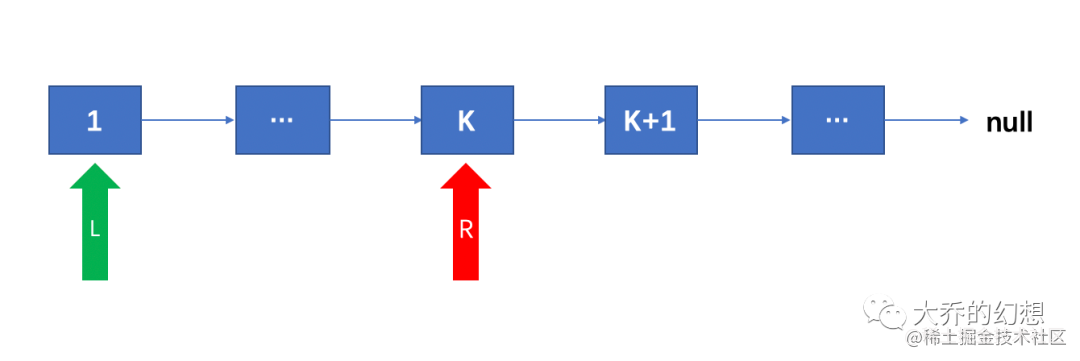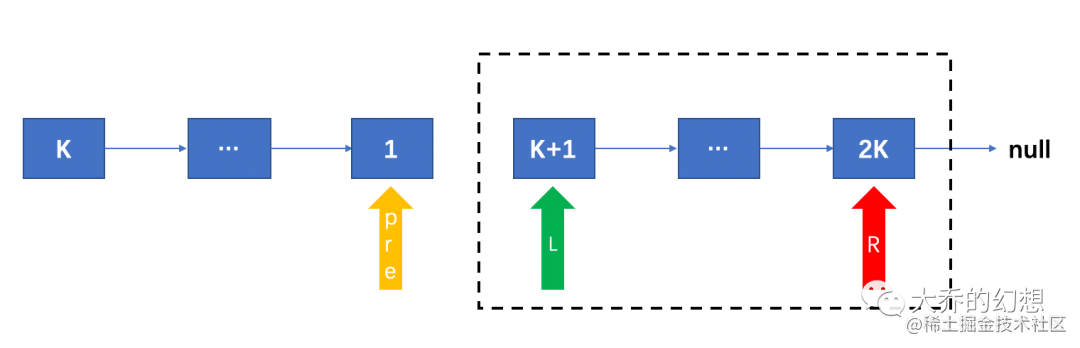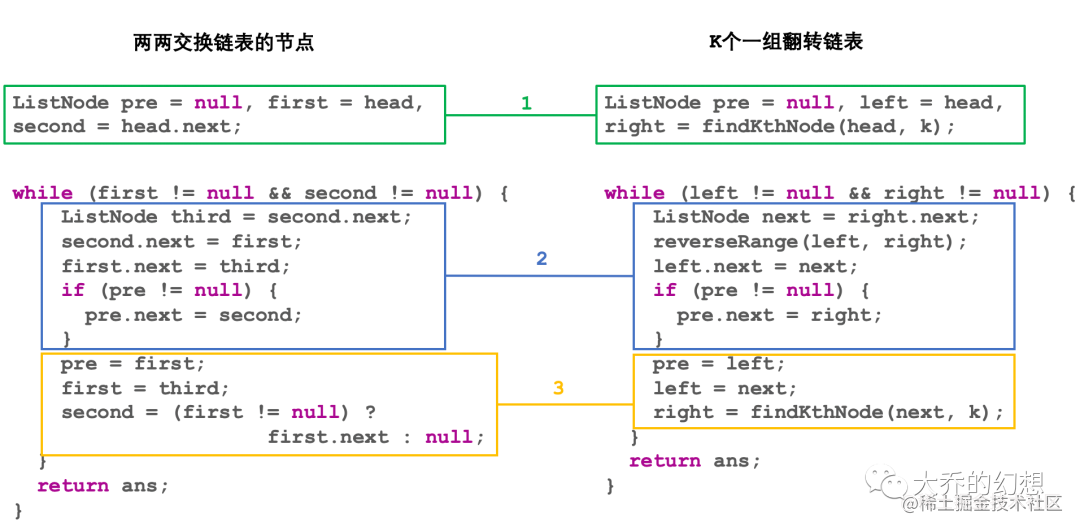# 链表反转全家桶（三）：动画详解两两交换链表中的节点

k 是一个正整数，它的值小于或等于链表的长度。``````private ListNode findKthNode(ListNode p, int k) {
int curCount = 0;
while (curCount < k - 1 && p != null) {
p = p.next;
curCount++;
}
return (curCount == k - 1) ? p : null;
}

`````` private void reverseRange(ListNode left, ListNode right) {
if (left == right) {
return;
}
ListNode exit = right.next;
ListNode cur = left, pre = null;
while (cur != exit) {
ListNode next = cur.next;
cur.next = pre;
pre = cur;
cur = next;
}
}``````class Solution {
public ListNode reverseKGroup(ListNode head, int k) {
}
if (right == null) {
}
ListNode ans = right;
while (left != null && right != null) {
ListNode next = right.next;
reverseRange(left, right);
left.next = next;
if (pre != null) {
pre.next = right;
}
pre = left;
left = next;
right = findKthNode(next, k);
}
return ans;
}

private void reverseRange(ListNode left, ListNode right) {
if (left == right) {
return;
}
ListNode exit = right.next;
ListNode cur = left, pre = null;
while (cur != exit) {
ListNode next = cur.next;
cur.next = pre;
pre = cur;
cur = next;
}
}

private ListNode findKthNode(ListNode p, int k) {
int curCount = 0;
while (curCount < k - 1 && p != null) {
p = p.next;
curCount++;
}
return (curCount == k - 1) ? p : null;
}
}• 时间复杂度：`O(n)`
• 空间复杂度：`O(1)`

``````class Solution {
public ListNode reverseKGroup(ListNode head, int k) {
if (right == null) {
return left;
}
ListNode next = reverseKGroup(right.next, k);
reverseRange(left, right);
left.next = next;
return right;
}

private void reverseRange(ListNode left, ListNode right) {
if (left == right) {
return;
}
reverseRange(left.next, right);
left.next.next = left;
left.next = null;
}

private ListNode findKthNode(ListNode p, int k) {
int curCount = 0;
while (curCount < k - 1 && p != null) {
p = p.next;
curCount++;
}
return (curCount == k - 1) ? p : null;
}
}

• 时间复杂度：`O(n)`
• 空间复杂度：`O(n)`，使用了递归，递归的栈深度为`n`